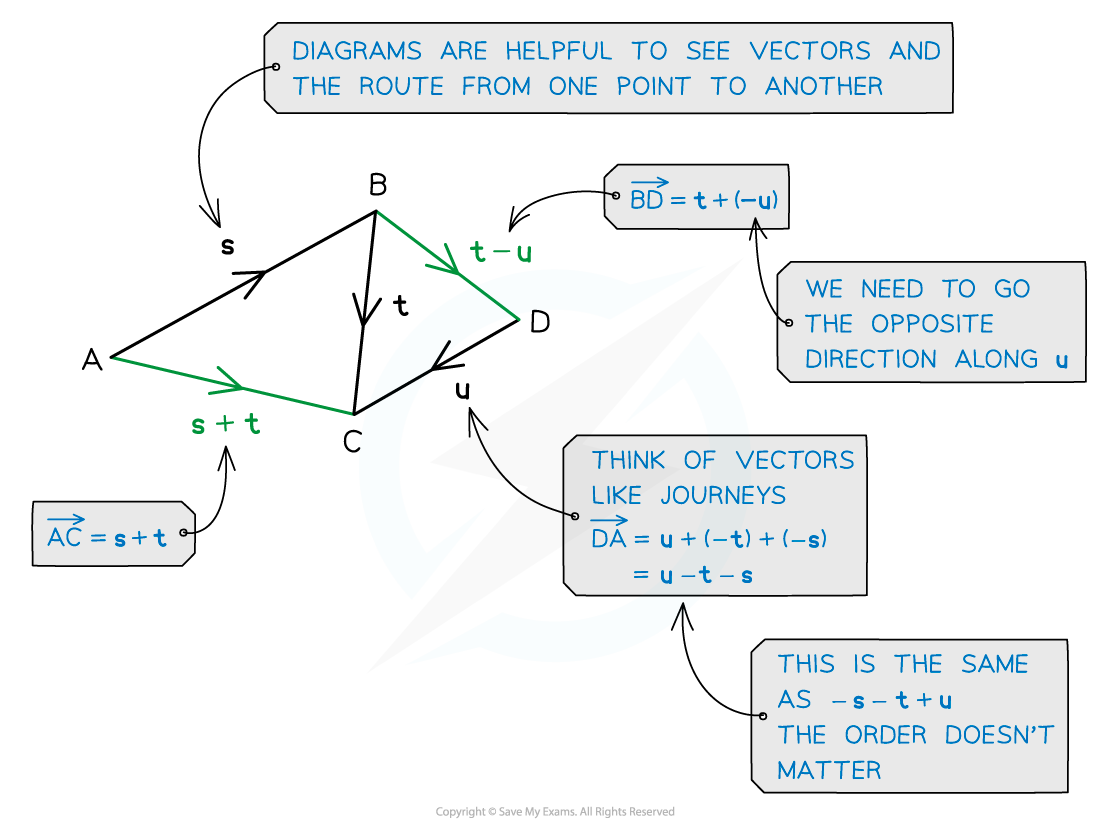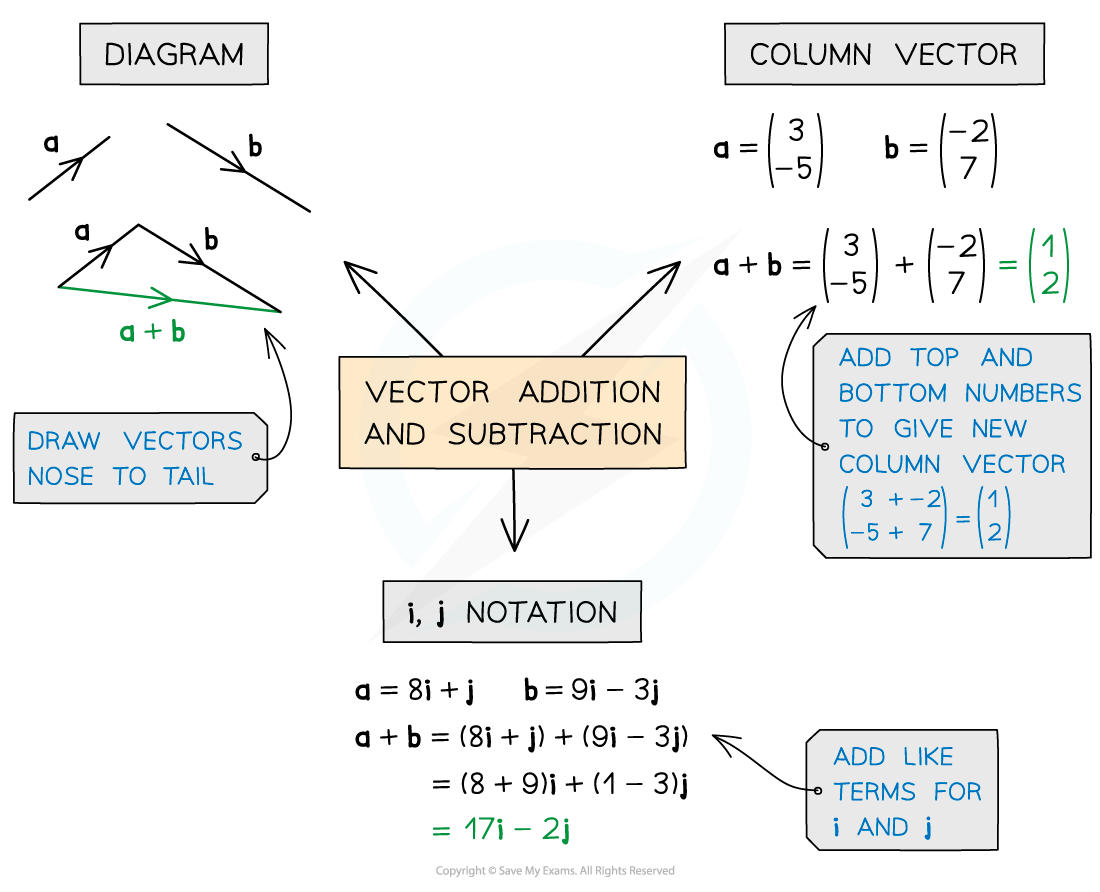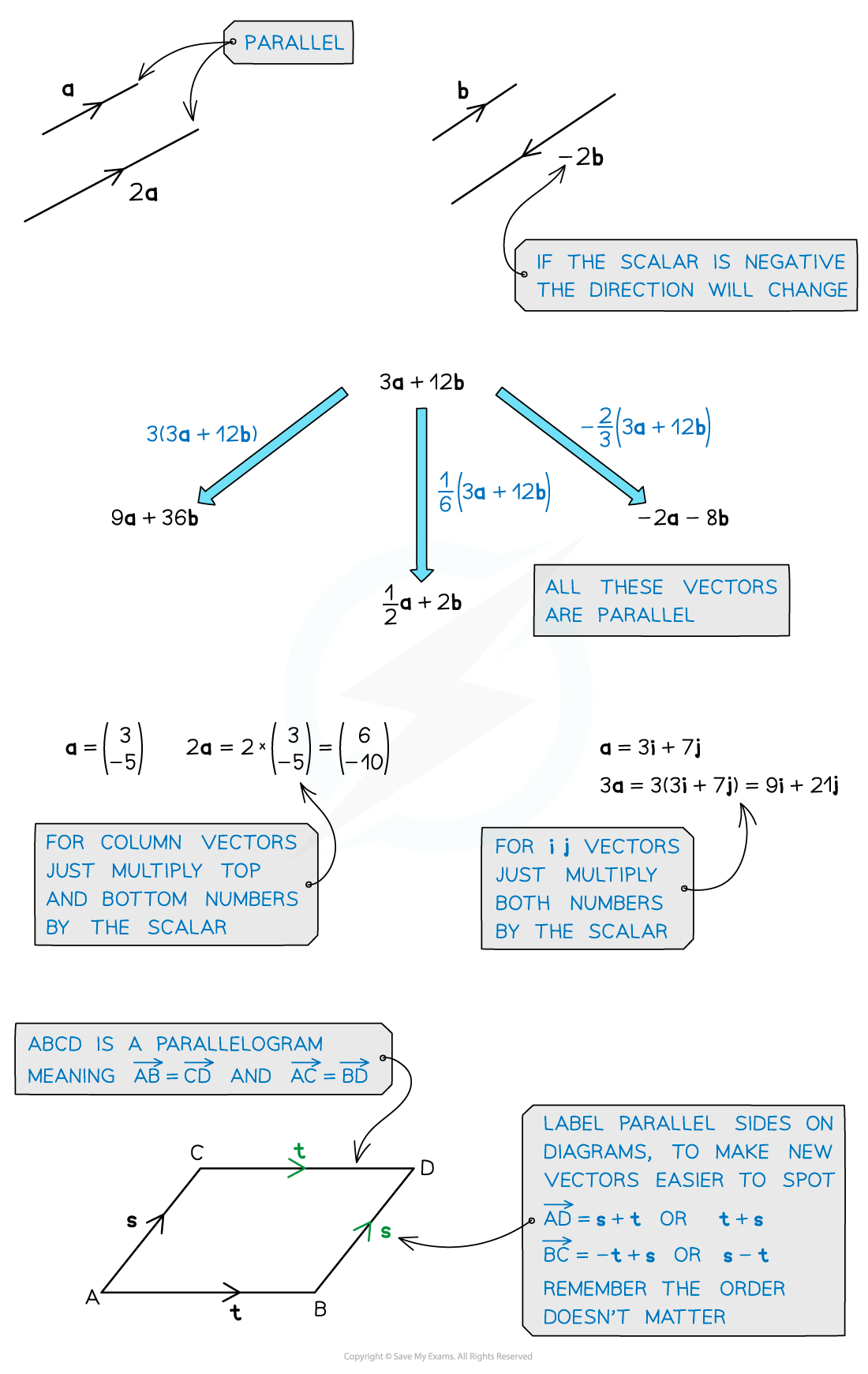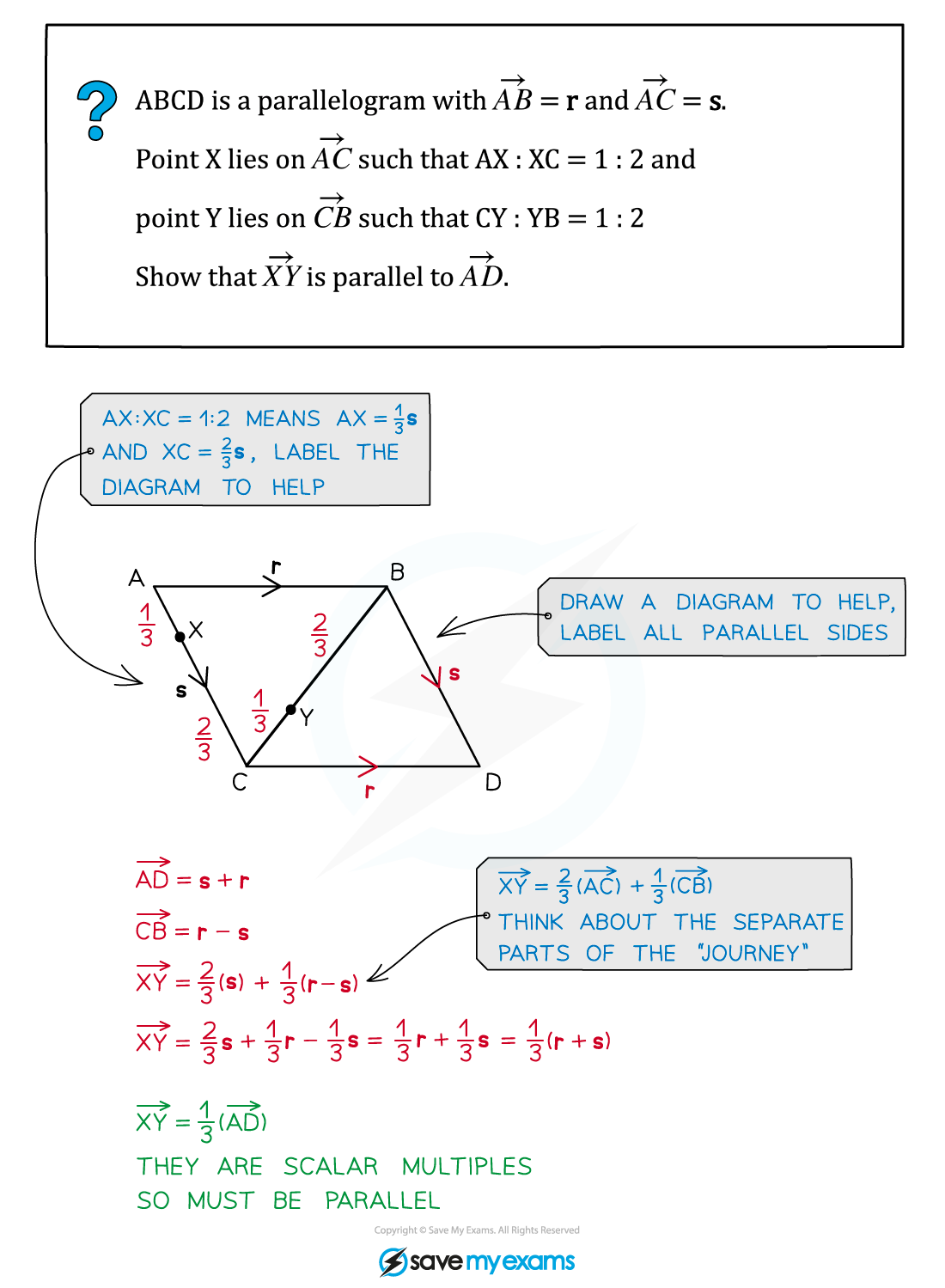# AQA A Level Maths: Pure复习笔记11.1.3 Vector Addition

• Adding vectors together lets us describes the movement between two points
• The single vector this creates is called the resultant vector
• Subtracting a vector is the same as adding the negative vector
• Adding the vectors PQ and QP gives the zero vector, denoted by a bold zero 0• You can add and subtract vectors in any of the forms previously described:#### Scalars and parallel vectors

• Multiplying by a positive scalar only changes the size of a vector, not its direction
• Two vectors are parallel if and only if one is a scalar multiple of the other#### Exam Tip

• Think of vectors like a journey from one place to another – you may have to take a detour eg. A to B might be A to O then O to B.
• Diagrams can help, so if there isn’t one, draw one. If there are any, labelling parallel vectors will help.

#### Worked Example